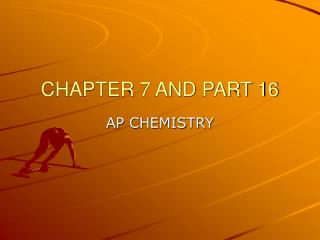DownloadDownload PresentationCHAPTER 7 AND PART 16

# CHAPTER 7 AND PART 16

Télécharger la présentation## CHAPTER 7 AND PART 16

- - - - - - - - - - - - - - - - - - - - - - - - - - - E N D - - - - - - - - - - - - - - - - - - - - - - - - - - -
##### Presentation Transcript

1. CHAPTER 7 AND PART 16 AP CHEMISTRY

2. LIGHT • Wavelength = λ • nm/ wave, m/wave, or nm or m • λf= c; c = 2.998 x 108 m/s • Frequency = f • Waves/s or 1/s = c/ λ • High frequency = short wavelength • Low frequency = long wavelength

3. E = hf Planck’s constant h = 6.626 x 10-34 J.s Continuous spectrum All wavelengths Line spectrum Discrete energies light going through a prism ROY G BIV PHOTON ENERGY

4. HYDROGEN ATOM • Bohr model used hydrogen atom • Electron orbits around nucleus • Energy levels: • Zero energy • where a photon and electron are in completely separate energy states within an atom • Ground state • lowest amount of energy an electron has • Excited state • electron getting extra energy • E = hf = Ehi - Elo • Electrons can only give off certain energies

5. QUANTUM NUMBERS • Most probable place an electron can be found • Ψ amplitude (height) of the electron wave • Ψ2 directly proportional to the probability of finding the electron • Quantum numbers • Principal energy level • n = 1, 2, 3, ..... etc. • Sublevels • s, p, d, and f • l = 0, 1, 2, 3, ...... etc.

6. Orbitals or orientations m = l to -l l = 0 then m = 0 l = 1 then m = 1, 0, -1 l = 2 then m = 2, 1, 0, -1, -2 Spin s = +1/2 , -1/2 Pauli Exclusion Principle No two electrons can have the same four quantum numbers Capacities Each energy level (n) has n sublevels Each sublevel (l) has 2l + 1 orbitals Each orbital (m) has 2 electrons CONTINUE

7. CONTINUE • Heisenberg principle • state that there are limitations in knowing what the position and momentum are at any given time • Probability distribution intensity of color -electron density map • Most probable place to find a hydrogen electron is .529Ǻ from nucleus • 1Ǻ = 10-10 m

8. QUANTUM NUMBERS • Polyelectronic atoms • Treat electrons as if they have nuclear attraction and average repulsion from other electrons • Effective nuclear charge • Zeff = Zactual - (electron repulsion) Z = atomic number • Periodic table can predict the filling of the sublevels • Elements in groups • Elements in groups • Transition metals in groups • The two sets of 14 elements at the bottom of the table fill

9. ORBITAL DIAGRAM • Hund’s rule • Within a given sublevel, the order of filling is such that there is the maximum number of half-filled orbitals • Monoatomic ion electron configuration • Ions with noble gas configuration • Anions are formed from atoms with • Cations are formed from atoms with

10. PERIODIC TRENDS • Atomic radius • In general atomic radii • Decreases as you move left to right • Increases as you move down • Effective nuclear charge • Zeffective = Z - S • Z = number or protons • S = number of core electrons that are shielding the outer electrons from the nucleus

11. IONIC RADIUS • Ionic radius • Ionic radius increases as you move down the group • Cations decrease left to right • Anions decrease left to right

12. IONIZATION ENERGY • Energy required to remove an electron • Increase as you move across left to right • Decrease as you move down

13. ELECTRONEGATIVITY • Electron Affinity • Energy released from an atom as it acquires another electron • Atomic radius • Decreases going across because the valence electrons are being pulled in, due to an increase of proton attraction. Shielding remains constant across the period • Electronegativity • How much an atom wants an electron

14. METALS • Metals • Metallic luster, ductile, malleable, good conductor of heat and electricity • Compounds formed from metals and nonmetals tend to be ionic

15. METALS AND NONMETALS • Nonmetals • No luster, poor conductors • Nonmetals tend to gain electrons to become anions • When compounds are made up chiefly of nonmetals they are molecular compounds • Most nonmetal oxides are • Semimetals • Some properties of both, brittle, semi-conductor • Trends in metallic characteristics • The more an element shows physical and chemical properties characteristic for metals, increase right to left and top to bottom